Author: Oscar Cronquist Article last updated on December 23, 2022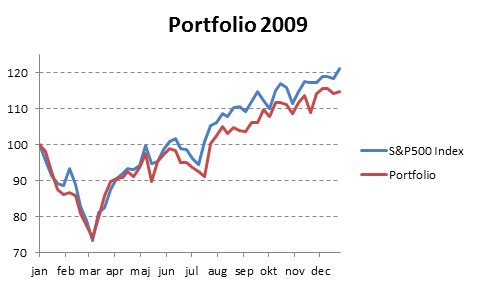By comparing your stock portfolio performance to index S&P500 you know if the time you spent on analyzing companies paid off. In fact, Warren Buffet recommends investing in an SP500 index fund if you have no knowledge of investing in the stock market. Let's see if your portfolio beats S&P500 over time.

In previous posts a few years ago I explained how to use NAV units to calculate stock portfolio performance. I am going to use Net Asset Value based on units for a fictional stock portfolio in this article.

### Get data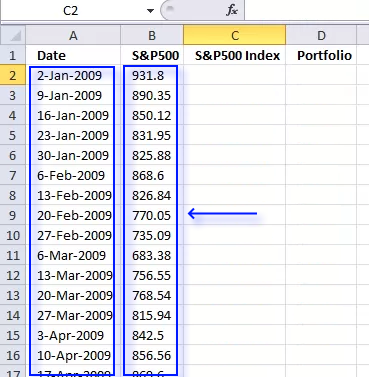We need monthly data from both the stock portfolio and the S&P 500. First get historical weekly prices from Yahoo Finance. Copy and paste dates and prices to a new sheet in your workbook.

### Calculate stock portfolio performance

The Excel workbook attached to Automate net asset value (NAV) calculation on your stock portfolio (vba) can automatically calculate portfolio performance using NAV (net asset value). You can't base portfolio perfomance on account balance.

Open the Excel workbook and insert the last date to each week and then press "Update" button. Make sure dates (col A) are sorted.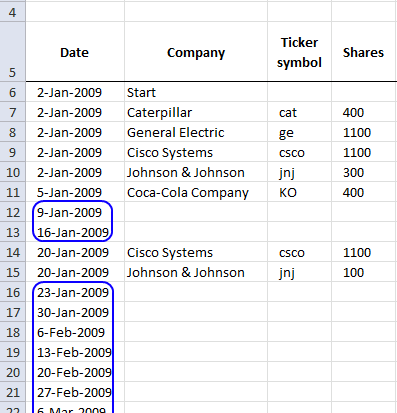### Copy values to a new table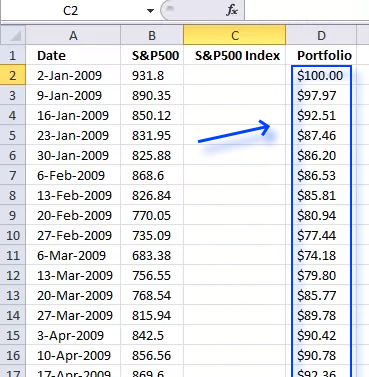1. Copy "NAV per share" data from the "Transactions" sheet and corresponding dates.
2. Select the new sheet containing the yahoo S&P 500 prices you geted.
3. Paste "NAV per share" data and corresponding dates.

### Indexing S&P 500 data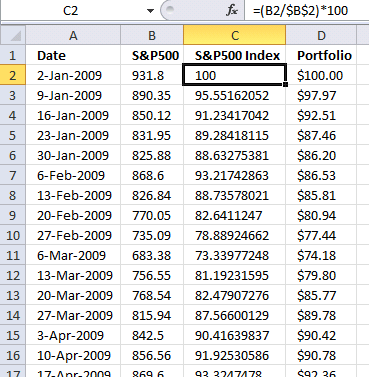Index SP500 to 100. This makes it a lot easier to compare performance relative to your stock portfolio. Both your stocks and the index must start at the same value, I am going for 100 which is often used.

Formula in cell C2:

=(B2/\$B\$2)*100

Copy formula to cells below as far as needed.

### Explaining formula in cell C2

#### Step 1 - Divide first value with current value

The forward slash is an arithmetic operator that lets you divide a number with another number. The first cell reference B2 is a relative cell reference that changes when you copy the cell and paste to cells below.

The second cell reference is an absolute cell reference that is locked to cell B2 all the time (except if you insert rows or columns). This allows us to divide the corresponding value on the same row with the first value and calculating the outcome in percentage date by date.

B2/\$B\$2

becomes

931.8/931.8

and returns 1.

#### Step 2 - Multiply with 100

The parentheses let you control the order of operations, we want it to do the division before we multiply with 100.

(B2/\$B\$2)*100

becomes

1*100

and returns 100.

### Create a chart showing portfolio and S&P 500 performance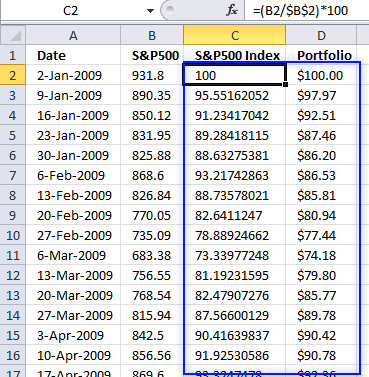1. Select values in column C and D.
2. Go to tab "Insert" on the ribbon.
3. Press with left mouse button on "Line chart" button.

### Get the Excel fileStock-portfolio-performance-chart.xls